# Problem A

Nine Knights

In the game of chess, knights are unique due to their “L-shaped” movement. A knight can move, as shown in Figure A.1, by either moving two squares sideways and one square up or down, or moving one square sideways and two squares either up or down.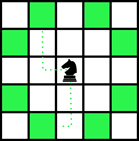Figure A.1: The highlighted squares show all possible moves for a knight.

In the Nine Knights puzzle, exactly nine knights must be positioned on a 5-by-5 board so that no knight can attack another knight with a single move. The configuration shown in Figure A.2 is an invalid solution because two of the knights can attack each other, where the configuration shown in Figure A.3 is a valid solution.

Figure A.2: Invalid game configuration                                         Figure A.3: Valid game configuration

Given the description of a game configuration, your job is to determine whether or not it represents a valid solution to the Nine Knights puzzle.

## Input

The input will consist of 5 lines, each having 5 characters. All characters will be either ’k’, indicating the placement of a knight, or ’.’, indicating an empty space on the board.

ACM-ICPC 2017 Mid-Central Regional Problem A: Nine Knights                                                            1

## Output

Display the word valid if the given chess board is a valid solution to the Nine Knights puzzle. Otherwise, display the word invalid.

Sample Input 1                                                                         Sample Output 1

 ...k. ...k. k.k.. .k.k. k.k.k invalid

Sample Input 2                                                                         Sample Output 2

 ..... ...k. k.k.k .k.k. k.k.k valid

Sample Input 3                                                                         Sample Output 3

 ..... ...k. k.k.k .k.k. k...k invalid

ACM-ICPC 2017 Mid-Central Regional Problem A: Nine Knights

题目大意 ： 输入一个5 * 5的矩阵， 其中有9个骑士， 他们是按照L型攻击，只是不会像中国象棋的马会被蹩马腿， 输出该矩阵是否满足各个骑士之间不会相互攻击

Accepted code：

#include<bits/stdc++.h>
using namespace std;

#define sc scanf
#define ls rt << 1
#define rs ls | 1
#define Min(x, y) x = min(x, y)
#define Max(x, y) x = max(x, y)
#define ALL(x) (x).begin(),(x).end()
#define SZ(x) ((int)(x).size())
#define MEM(x, b) memset(x, b, sizeof(x))
#define lowbit(x) ((x) & (-x))
#define P2(x) ((x) * (x))

typedef long long ll;
const int MAXN = 1e5 + 10;
const int INF = 0x3f3f3f3f;

string s;
bool flag;
int ans;
int dir = { 2, 1, 1, 2, -1, 2, -2, 1, -2, -1, -1, -2, 1, -2, 2, -1 };
bool dfs(int x, int y) {
for (int i = 0; i < 8; i++) {
int xx = x + dir[i];
int yy = y + dir[i];
if (xx >= 0 && yy >= 0 && xx < 5 && yy < 5) {
if (s[xx][yy] == 'k') return true;
}
}
return false;
}

int main()
{
for (int i = 0; i < 5; i++) {
cin >> s[i];
for (int j = 0; j < 5; j++) {
if (s[i][j] == 'k') ans++;
}
}
if (ans != 9) cout << "invalid" << endl; // 满足9个骑士
else {
for (int i = 0; i < 5; i++) {
for (int j = 0; j < 5; j++) {
if (s[i][j] == 'k' && dfs(i, j)) {
flag = 1; // 一个会被攻击即退出
break;
}
}
}
if (flag) cout << "invalid" << endl;
else cout << "valid" << endl;
}
return 0;
}

# Problem B

Batter Up

While the Chicago Cubs were ecstatic with their 2016 World Series championship, they were eliminated from the playoffs in 2017. Looking ahead to 2018 they are beginning to embrace the more data-driven analysis of player’s values known as Sabermetrics.

For example, a player’s batting average is calculated by dividing the total number of base hits by the total number of official at-bats. One limitation of using the batting average to evaluate players is that it treats all hits equally, rather than taking into account doubles, triples or home runs. For this reason, analysts often prefer to consider what is known as the slugging percentage, which distinguishes between different hit outcomes. To calculate the slugging percentage, the total number of bases of all hits is divided by the total numbers of time at bat, that did not result in walks, or at-bats.

More specifically, an at-bat can earn 0, 1, 2, 3 or 4 bases (these are referred to as official at-bats). Furthermore, some at-bats, such as those that result in a base-on-balls (i.e., a “walk”) are not considered in either the player’s batting average or slugging percentage.

For example, if a player hits a triple (3 bases), strikes out (0 bases), and hits a double (2 bases), their slugging percentage would be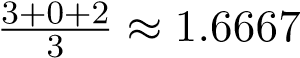. If a player hits a single (1 base), walks, and hits a home run (4 bases), the slugging level would be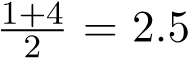. Notice that in this case, the denominator is two, not three, because the walk does not count towards the slugging percentage.

## Input

The input is composed of two lines. The first line contains a single positive integer n (1 ≤ n ≤ 100) that specifies the number of at-bats. The second line contains n integers, separated by spaces, each describing one of those at-bats. Strike-outs, singles, doubles, triples, and home runs are represented as 0, 1, 2, 3, 4, respectively. Walks are represented as -1. You may assume that there will always be at least one official at-bat (i.e., at least one at-bat will not be a walk).

## Output

Display the player’s slugging percentage as a real number, accurate to within an absolute or relative error of 10−3. We recommend that you do not round the value that you calculate.

Sample Input 1                                                                         Sample Output 1

 3 3 0 2 1.6666666666666667 Sample Input 2 Sample Output 2 3 1 -1 4 2.5

ACM-ICPC 2017 Mid-Central Regional Problem B: Batter Up

Sample Input 3                                                                         Sample Output 3

 11 -1 -1 -1 -1 0 0 0 0 0 0 1 0.142857

ACM-ICPC 2017 Mid-Central Regional Problem B: Batter Up

题目大意 ： 花里胡哨的棒球规则就不说了，直接输出大于等于0的数之和再除以他们的个数

Accpted code

#include<bits/stdc++.h>
using namespace std;

#define sc scanf
#define ls rt << 1
#define rs ls | 1
#define Min(x, y) x = min(x, y)
#define Max(x, y) x = max(x, y)
#define ALL(x) (x).begin(),(x).end()
#define SZ(x) ((int)(x).size())
#define MEM(x, b) memset(x, b, sizeof(x))
#define lowbit(x) ((x) & (-x))
#define P2(x) ((x) * (x))

typedef long long ll;
const int MAXN = 1e5 + 10;
const int INF = 0x3f3f3f3f;

double p[MAXN], n, ans, m;

int main()
{
cin >> n;
for (int i = 0; i < n; i++) {
sc("%lf", &p[i]);
if (p[i] >= 0) ans += p[i], m++;
}
printf("%.11f\n", ans / m);
return 0;
}

# Problem D

No Duplicates

There is a game in which you try not to repeat a word while your opponent tries to see if you have repeated one.

"THE RAIN IN SPAIN" has no repeats.

"IN THE RAIN AND THE SNOW" repeats THE.

"THE RAIN IN SPAIN IN THE PLAIN" repeats THE and IN.

Write a program to test a phrase.

## Input

Input is a line containing words separated by single spaces, where a word consists of one or more uppercase letters. A line contains no more than 80 characters.

## Output

The output is "yes" if no word is repeated, and "no" if one or more words repeat.

Sample Input 1                                                                         Sample Output 1

 THE RAIN IN SPAIN yes Sample Input 2 Sample Output 2 IN THE RAIN AND THE SNOW no Sample Input 3 Sample Output 3 THE RAIN IN SPAIN IN THE PLAIN no

ACM-ICPC 2017 Mid-Central Regional Problem D: No Duplicates

Accpted code

#include<bits/stdc++.h>
using namespace std;

#define sc scanf
#define ls rt << 1
#define rs ls | 1
#define Min(x, y) x = min(x, y)
#define Max(x, y) x = max(x, y)
#define ALL(x) (x).begin(),(x).end()
#define SZ(x) ((int)(x).size())
#define MEM(x, b) memset(x, b, sizeof(x))
#define lowbit(x) ((x) & (-x))
#define P2(x) ((x) * (x))

typedef long long ll;
const int MAXN = 1e5 + 10;
const int INF = 0x3f3f3f3f;

string line;
set <string> st;
int j, ans;

int main()
{
getline(cin, line, '\n');
string s = "";
for (int i = 0; i < line.size(); i++) {
if (line[i] != ' ') s += line[i];
else {
ans++;
st.insert(s);
s = "";
j = i;
}
}
if (!ans) cout << "yes" << endl;
else {
st.insert(line.substr(j + 1, line.size()));
if (st.size() == ans + 1) cout << "yes" << endl;
else cout << "no" << endl;
}
return 0;
}

This page is intentionally left (almost) blank.

# Problem E

Honey Heist

0x67 is a scout ant searching for food and discovers a beehive nearby. As it approaches the honeycomb, 0x67 can sense an area inside packed with dried honey that can be easily carried back to the nest and stored for winter. However, it must burrow through the honeycomb to reach the cell containing the sweet loot. If 0x67 can create a passage to the honey to help the other ants find it, it will do so before returning to the nest. The cells of the honeycomb are numbered in row major order, so cell IDs can be assigned as shown below: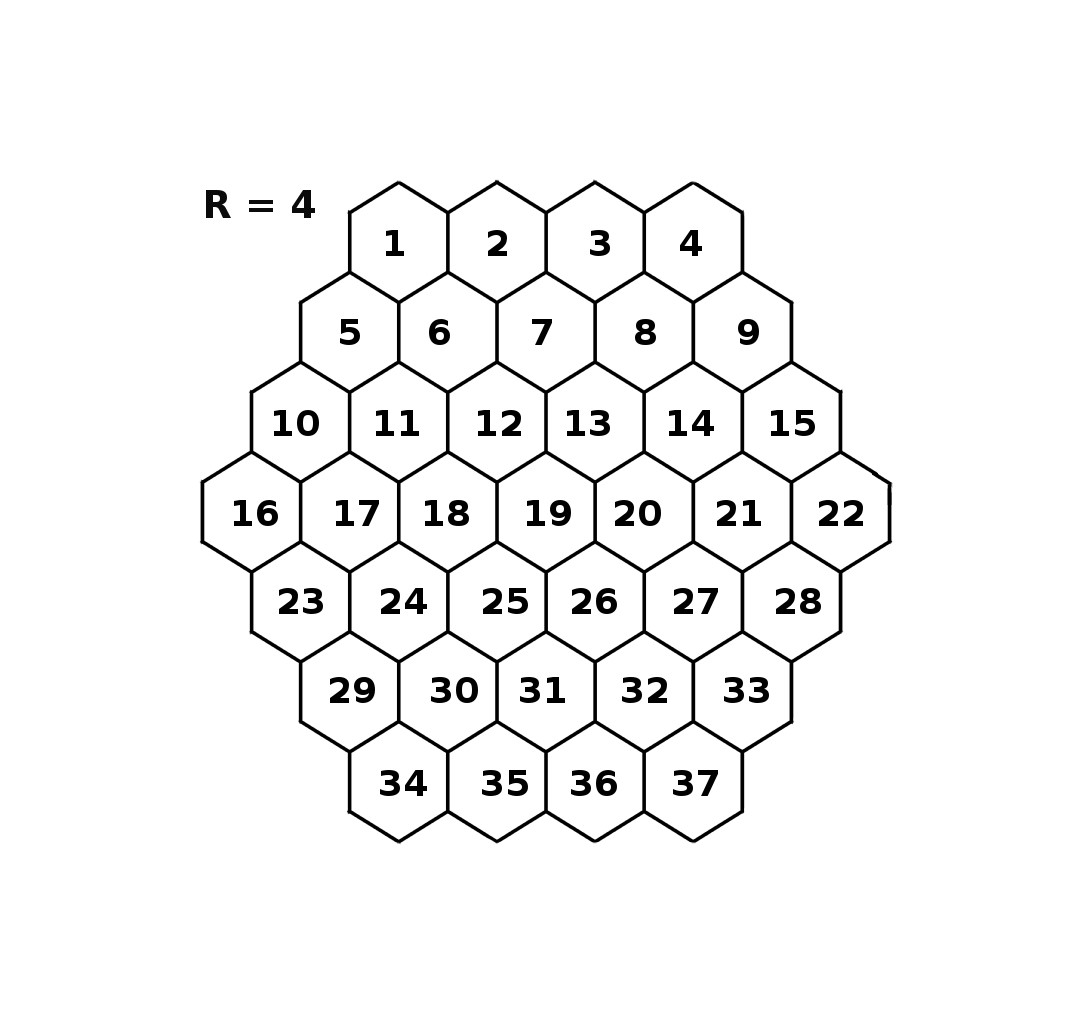When 0x67 discovers the opening to the honeycomb, it enters the cell. Some ants are stronger than others, depending on their age, so 0x67 can only chew through at most N cells before its jaw wears out and must return to the nest to recuperate. The honeycomb is hexagonal, and each edge length is R cells. 0x67 enters through a hole at location A and must get to the honey at location B by chewing a path through no more than N adjacent cells. Because ants can be competitive, 0x67 wants to reach the honey by chewing through the fewest possible cells. 0x67 can also sense some of the cells are hardened with wax and impossible to penetrate, so it will have to chew around those to reach the cell at location B.

Scout ants have rudimentary computational skills, and before 0x67 begins to chew, it will work out where it needs to go, and compute K, the least number of cells it needs to chew through to get from A to B, where B is the Kth cell. If K > N, 0x67 will not be strong enough to make the tunnel. When 0x67 returns to the nest, it will communicate to its nestmates how many cells it chewed through to get to B, or will report that it could not get to the honey.

## Input

The input contains two lines. The first line contains five blank separated integers: R N A B X

R: the length (number of cells) of each edge of the grid, where 2 ≤ R ≤ 20. The total number of cells in the grid can be determined by taking a difference of cubes, R3 − (R − 1)3.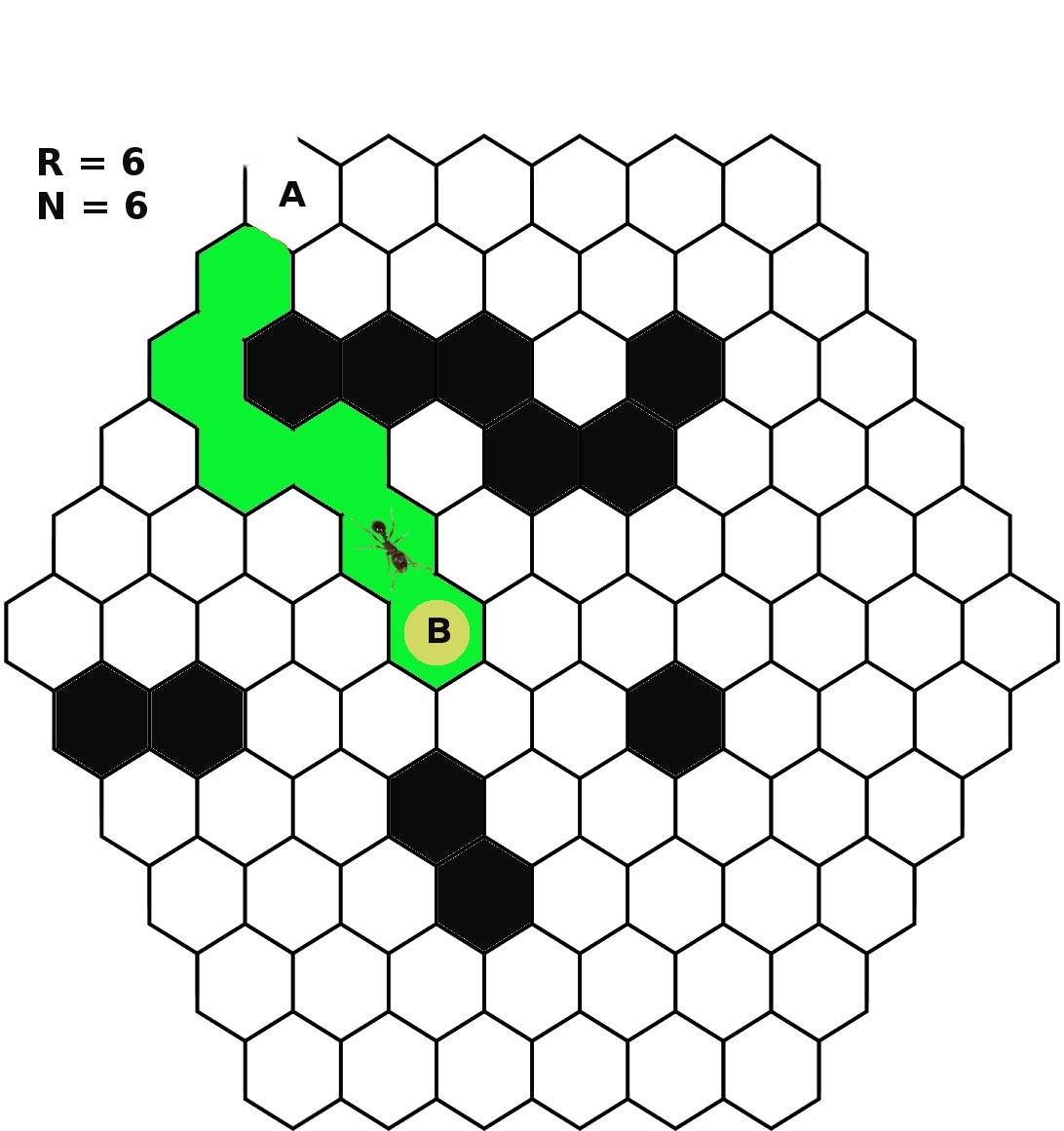Figure E.1: K=6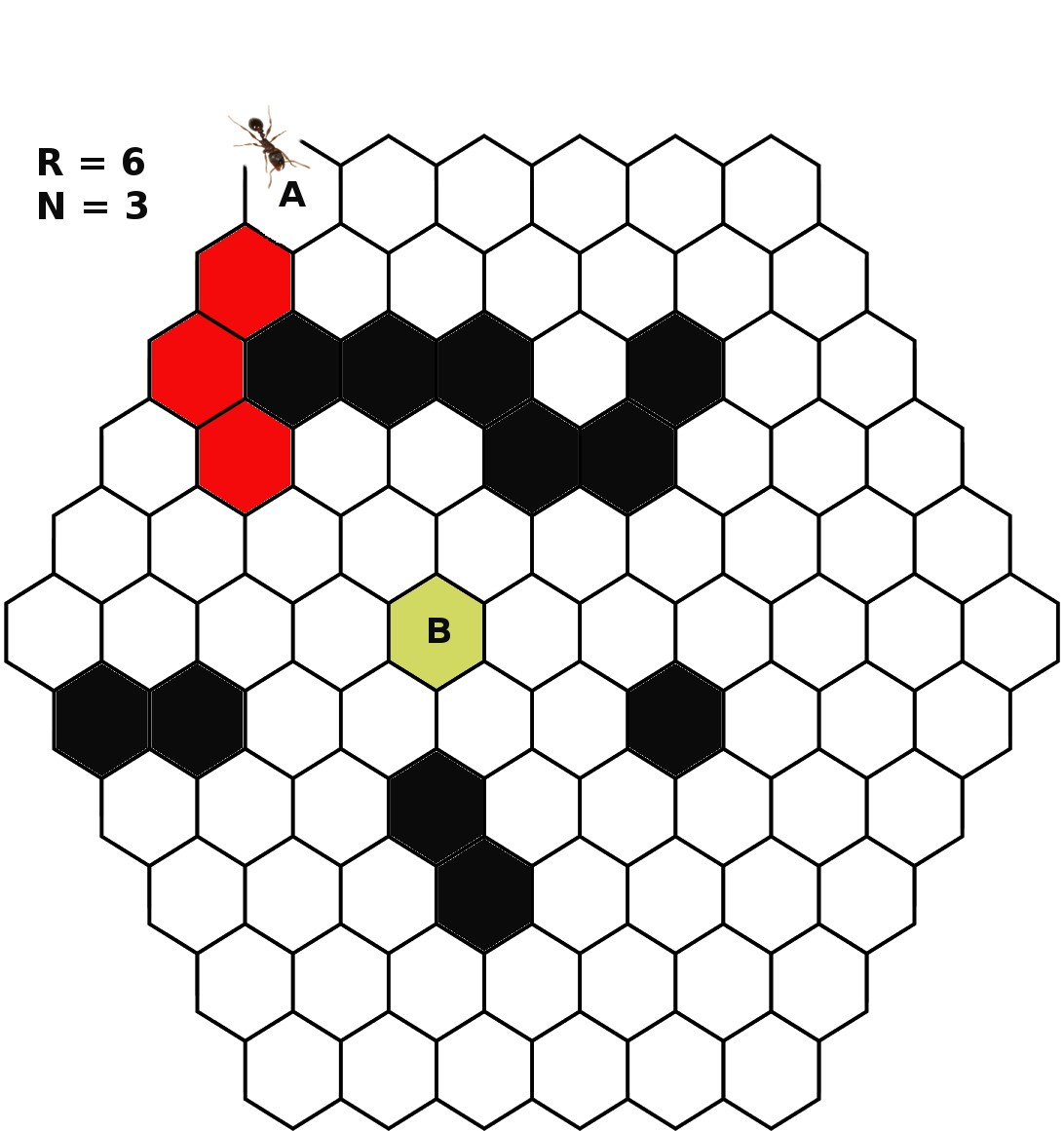Figure E.2: No

10

N: the maximum number of cells 0x67 can chew through, where 1 ≤ N < R3 − (R − 1)3.

A: the starting cell ID, This cell is located on one of the grid edges: The cell has fewer than six neighbors.

B: the cell ID of the cell containing the honey, where 1 ≤ B R3 − (R − 1)3.

X: the number of wax-hardened cells, where 0 ≤ X < (R3 − (R − 1)3) − 1.

The second line contains X integers separated by spaces, where each integer is the ID of a wax-hardened cell.

The ID’s, A, B, and all the ID’s on the second line, are distinct positive integers less than or equal to R3 − (R − 1)3.

## Output

A single integer K if 0x67 reached the honey at cell B, where B is the Kth cell, otherwise the string No if it was impossible to reach the honey by chewing through N cells or less.

Sample Input 1                                                                         Sample Output 1

 6 6 1 45 11 15 16 17 19 26 27 52 53 58 65 74 6 Sample Input 2 Sample Output 2 6 3 1 45 11 15 16 17 19 26 27 52 53 58 65 74 No

题目大意 ： 输入 R， N, A, B,  X 分别表示该蜂巢的边长， 蚂蚁最多可以走的深度，起点，终点，障碍物数目，以下X个数分别为各个障碍物的ID， 输出是否可以在N内到达终点，如果可以，输出最短路径， 否则输出 No

Accpeted code

#include<iostream>
#include<cstring>
#include<cstdio>
#include<queue>
using namespace std;

#define sc scanf
#define mem memset
#define Min(a, b) a = min(a, b)
#define Max(a, b) a = max(a, b)
typedef long long ll;
const int MAXN = 1e5 + 10;
const int INF = 0x3f3f3f3f;

struct Edge
{
int v, w, next;
}e[MAXN << 1];
struct node
{
int id, w;
bool operator < (const node &oth) const
{
return w > oth.w;
}
}mid;
int head[MAXN], n, m, cnt, T, X;
int dis[MAXN], val[MAXN], r, sp, tp, x;
bool vis[MAXN];
void init() {
mem(e, 0, sizeof(e));
cnt = 0;
}
void add(int from, int to, int dis) {
e[++cnt].v = to; e[cnt].w = dis;

e[++cnt].v = from; e[cnt].w = dis;
}
void dijkstra(int u) {
priority_queue <node> q;
mem(dis, INF, sizeof(dis));
dis[u] = 0;
q.push({ u, 0 });
while (!q.empty()) {
mid = q.top();
q.pop();
int ans = mid.id;
if (mid.w != dis[ans]) continue;
for (int i = head[ans]; i != -1; i = e[i].next) {
if (dis[e[i].v] > dis[ans] + e[i].w) {
dis[e[i].v] = dis[ans] + e[i].w;
q.push({ e[i].v, dis[e[i].v] });
}
}
}
} // 以上都是套板子

int main()
{
cin >> r >> n >> sp >> tp >> x; // 半径， 深度， 起点， 终点， 障碍物个数
init();
m = r * r * r - (r - 1) * (r - 1) * (r - 1); // 记录最大的数字
for (int i = 0; i < x; i++) {
int t; sc("%d", &t);
vis[t] = 1;  // 标记障碍物
}
for (int i = 1; i <= r - 1; i++) { // 预处理把层数表示出来
for (int j = 1; j < i + r; j++) {
X++;
val[X] = val[m - X] = i - 1;
}
}
for (int i = X + 1; i <= X + 2 * r - 1; i++) val[i] = r - 1; // 接上预处理
int cnt = r, tot = 1;
for (int i = 1; i < r; i++) { // 开始建图， 除了最右边的点， 都有三个点与之相连
for (int j = 1; j < cnt; j++) {
int ui = tot + 1, vi = tot + val[tot] + r;
int ci = tot + val[tot] + r + 1;
if (vis[tot] || vis[ui]) add(tot, ui, INF);
else add(tot, ui, 1);
if (vis[tot] || vis[vi]) add(tot, vi, INF);
else add(tot, vi, 1);
if (vis[tot] || vis[ci]) add(tot, ci, INF);
else add(tot, ci, 1);
tot++;
} // 下面是特判最右边的点
int ui = tot + val[tot] + r, vi = tot + val[tot] + r + 1;
if (vis[tot] || vis[ui]) add(tot, ui, INF);
else add(tot, ui, 1);
if (vis[tot] || vis[vi]) add(tot, vi, INF);
else add(tot, vi, 1);
cnt++, tot++;
} // 到中间截至
cnt = r, tot = m;
for (int i = r - 1; i >= 1; i--) { // 下半部分与上面几乎一模一样
for (int j = 1; j < cnt; j++) {
int ui = tot - 1, vi = tot - r - val[tot];
int ci = tot - val[tot] - r - 1;
if (vis[tot] || vis[ui]) add(tot, ui, INF);
else add(tot, ui, 1);
if (vis[tot] || vis[vi]) add(tot, vi, INF);
else add(tot, vi, 1);
if (vis[tot] || vis[ci]) add(tot, ci, INF);
else add(tot, ci, 1);
tot--;
} // 特判
int ui = tot - val[tot] - r, vi = tot - val[tot] - r - 1;
if (vis[tot] || vis[ui]) add(tot, ui, INF);
else add(tot, ui, 1);
if (vis[tot] || vis[vi]) add(tot, vi, INF);
else add(tot, vi, 1);
cnt++, tot--;
}
dijkstra(sp);
if (dis[tp] <= n) cout << dis[tp] << endl;
else cout << "No" << endl;
}

This page is intentionally left (almost) blank.

# Problem F

Orderly Class

Ms. Thomas is managing her class of n students.

She placed all her students in a line, and gave the i-th student from the left a card with the letter ai written on it.

She would now like to rearrange the students so that the i-th student from the left has a card with the letter bi written on it.

To do this, she will choose some consecutive group of students, and reverse their order. Students will hold on to their original cards during this process.

She’s now wondering, what is the number of valid ways to do this? (It may be impossible, in which case, the answer is zero).

With sequences abba and aabb, Ms. Thomas can choose the group a(bba). With sequences caxcab and cacxab, Ms. Thomas can choose ca(xc)ab or c(axca)b. With sequences a and z, there are clearly no solutions.

## Input

The input is two lines of lowercase letters, A and B. The i-th character of A and B represent ai and bi respectively. It is guaranteed that A and B have the same positive length, and A and B are not identical. The common length is allowed to be as large as 100000.

## Output

For each test case, output a single integer, the number of ways Ms. Thomas can reverse some consecutive group of A to form the line specified by string B.

Sample Input 1                                                                         Sample Output 1

 abba aabb 1 Sample Input 2 Sample Output 2 caxcab cacxab 2 Sample Input 3 Sample Output 3 a z 0

ACM-ICPC 2017 Mid-Central Regional Problem F: Orderly Class

Accepted code

#include<bits/stdc++.h>
using namespace std;

#define sc scanf
#define ls rt << 1
#define rs ls | 1
#define Min(x, y) x = min(x, y)
#define Max(x, y) x = max(x, y)
#define ALL(x) (x).begin(),(x).end()
#define SZ(x) ((int)(x).size())
#define MEM(x, b) memset(x, b, sizeof(x))
#define lowbit(x) ((x) & (-x))
#define P2(x) ((x) * (x))

typedef long long ll;
const int MAXN = 1e5 + 10;
const int INF = 0x3f3f3f3f;

string a, b;
int l, r;

int main()
{
while (cin >> a >> b){
for (int i = 0; i < a.size(); i++) {
if (a[i] != b[i]) { l = i; break; }
}
for (int i = a.size() - 1; i >= 0; i--) {
if (a[i] != b[i]) { r = i; break; }
} // 找到最大有差异的区间
string s1 = a.substr(l, r - l + 1), s2 = b.substr(l, r - l + 1);
reverse(ALL(s1)); // 反转看是否相同
if (s1 != s2) { cout << 0 << endl, exit(0); }
int ans = 1;
l--; r++; // 区间以外的字符一一对应
while (l >= 0 && r < a.size() && a[l] == a[r]) {
l--; r++;
ans++;
}
cout << ans << endl;
}
return 0;
}

# Problem G

Faulty Robot

As part of a CS course, Alice just finished programming her robot to explore a graph having n nodes, labeled 1,2,...,n, and m directed edges. Initially the robot starts at node 1.

While nodes may have several outgoing edges, Alice programmed the robot so that any node may have a forced move to a specific one of its neighbors. For example, it may be that node 5 has outgoing edges to neighbors 1, 4, and 6 but that Alice programs the robot so that if it leaves 5 it must go to neighbor 4.

If operating correctly, the robot will always follow forced moves away from a node, and if reaching a node that does not have a forced move, the robot stops. Unfortunately, the robot is a bit buggy, and it might violate those rules and move to a randomly chosen neighbor of a node (whether or not there had been a designated forced move from that node). However, such a bug will occur at most once (and might never happen).

Alice is having trouble debugging the robot, and would like your help to determine what are the possible nodes where the robot could stop and not move again.

We consider two sample graphs, as given in Figures G.1 and G.2. In these figures, a red arrow indicate an edge corresponding to a forced move, while black arrows indicate edges to other neighbors. The circle around a node is red if it is a possible stopping node.

Figure G.1: First sample graph.                                                Figure G.2: Second sample graph.

In the first example, the robot will cycle forever through nodes 1, 5, and 4 if it does not make a buggy move. A bug could cause it to jump from 1 to 2, but that would be the only buggy move, and so it would never move on from there. It might also jump from 5 to 6 and then have a forced move to end at 7.

In the second example, there are no forced moves, so the robot would stay at 1 without any buggy moves. It might also make a buggy move from 1 to either 2 or 3, after which it would stop.

## Input

The first line contains two integers n and m, designating the number of nodes and number of edges such that 1 ≤ n ≤ 103, 0 ≤ m ≤ 104. The next m lines will each have two integers a and b, 1 ≤ |a|,b n and |a| 6= b. If a > 0, there is a directed edge between nodes a and b that is not forced. If a < 0, then there is a forced directed edge from −a to b. There will be at most 900 such forced moves. No two directed edges will be the same. No two starting nodes for forced moves will be the same.

ACM-ICPC 2017 Mid-Central Regional Problem G: Faulty Robot

## Output

Display the number of nodes at which the robot might come to a rest.

Sample Input 1                                                                         Sample Output 1

 7 9 2 3 -1 5 2 6 5 1 -4 1 5 6 -6 7 -5 4 2

Sample Input 2                                                                         Sample Output 2

 3 2 1 2 1 3 3

ACM-ICPC 2017 Mid-Central Regional Problem G: Faulty Robot

题目大意 ： 一个机器人按照红色箭头指向的方向行动， 但是他有一次“叛变”的机会， 会按照黑色线路走。整个过程直到他再也没有红色线路可走为止， 输出机器人可能停止的位置的个数，通过第二个样例可以知道， 机器人也可以不“叛变”

Accepted code

#include<iostream>
#include<cstring>
#include<cstdio>
#include<queue>
using namespace std;

#define sc scanf
#define mem memset
#define Min(a, b) a = min(a, b)
#define Max(a, b) a = max(a, b)
typedef long long ll;
const int MAXN = 1e5 + 10;
const int INF = 0x3f3f3f3f;

struct Edge
{
int v, w, next;
}e[MAXN << 1];
struct node
{
int id, w;
bool operator < (const node &oth) const
{
return w > oth.w;
}
}mid;
int head[MAXN], n, m, cnt, T;
int dis[MAXN], dep[MAXN];
void init() {
mem(e, 0, sizeof(e));
cnt = 0;
}
void add(int from, int to, int dis) {
e[++cnt].v = to;
e[cnt].w = dis;
}
void dijkstra(int u) {
priority_queue <node> q;
mem(dis, INF, sizeof(dis));
dis[u] = 0;
q.push({ u, 0 });
while (!q.empty()) {
mid = q.top();
q.pop();
int ans = mid.id;
if (mid.w != dis[ans]) continue;
for (int i = head[ans]; i != -1; i = e[i].next) {
if (dis[e[i].v] > dis[ans] + e[i].w) {
dis[e[i].v] = dis[ans] + e[i].w;
q.push({ e[i].v, dis[e[i].v] });
}
}
}
}

int main()
{
cin >> n >> m;
init();
for (int i = 0; i < m; i++) {
int ui, vi;
sc("%d %d", &ui, &vi);
if (ui < 0) {  // 红色线路
dep[-ui]++;  // 标记
}
else add(ui, vi, 1);
}
dijkstra(1);
int ans = 0;
for (int i = 1; i <= n; i++) {
if (dis[i] <= 1 && dep[i] == 0) ans++; // 必须是黑色线路才可停止
}
cout << ans << endl;
return 0;
}

# Problem H

Hopscotch

You’re playing hopscotch! You start at the origin and your goal is to hop to the lattice point (N,N). A hop consists of going from lattice point (x1,y1) to (x2,y2), where x1 < x2 and y1 < y2.

You dislike making small hops though. You’ve decided that for every hop you make between two lattice points, the x-coordinate must increase by at least X and the y-coordinate must increase by at least Y .

Compute the number of distinct paths you can take between (0,0) and (N,N) that respect the above constraints. Two paths are distinct if there is some lattice point that you visit in one path which you don’t visit in the other.

Hint: The output involves arithmetic mod 109 + 7. Note that with p a prime like 109 + 7, and x an integer not equal to 0 mod p, then x(xp−2) mod p equals 1 mod p.

Input

The input consists of a line of three integers, N X Y . You may assume 1 ≤ X,Y N ≤ 106.

## Output

The number of distinct paths you can take between the two lattice points can be very large. Hence output this number modulo 1000000007 (109 + 7).

Sample Input 1                                                                         Sample Output 1

 2 1 1 2 Sample Input 2 Sample Output 2 7 2 3 9

ACM-ICPC 2017 Mid-Central Regional Problem H: Hopscotch

题目大意 ： 从坐标 （0， 0） 到左边 （n， n）， 每次x轴方向必须至少走x 步， y轴方向必须至少走y步， 输出共有多少种走法

Accepted code

#include<bits/stdc++.h>
using namespace std;

#define sc scanf
#define ls rt << 1
#define rs ls | 1
#define Min(x, y) x = min(x, y)
#define Max(x, y) x = max(x, y)
#define ALL(x) (x).begin(),(x).end()
#define SZ(x) ((int)(x).size())
#define MEM(x, b) memset(x, b, sizeof(x))
#define lowbit(x) ((x) & (-x))
#define P2(x) ((x) * (x))

typedef long long ll;
const int MOD = 1e9 + 7;
const int MAXN = 1e6 + 7;
inline ll qpow(ll a, ll b){ ll r = 1, t = a; while (b){ if (b & 1)r = (r*t) % MOD; b >>= 1; t = (t*t) % MOD; }return r; }
ll fac[MAXN], inv[MAXN];
ll n, x, y, ans;

ll init(){
fac = 1;
for (int i = 1; i < MAXN; i++)
fac[i] = fac[i - 1] * i % MOD;
inv[MAXN - 1] = qpow(fac[MAXN - 1], MOD - 2);
for (int i = MAXN - 2; i >= 0; i--)
inv[i] = inv[i + 1] * (i + 1) % MOD;
return 0;
}
ll C(ll n, ll m){
if (n < m) return 0;
return fac[n] * inv[m] % MOD * inv[n - m] % MOD;
}

int main()
{
init();
cin >> n >> x >> y;
ll m = min(n / x, n / y);
for (ll i = 1; i <= m; i++)
ans = (ans + C(n - i*(x - 1) - 1, i - 1) % MOD*C(n - i*(y - 1) - 1, i - 1) % MOD) % MOD;
cout << ans << endl;
return 0;
}